# Wine Quality Prediction Using k-NN Regressor

Data and its interpretation are helping many industries across the world. Machine learning models built over the data collected are solving some of the unsolved and challenging tasks. One of such tasks can be predicting the quality of wine along with some quantitative measurement. Judging the quality of Wine manually is really a tough task; even the professional Wine tasters have an accuracy of only 71%. Gaining the title of a Wine taster is a quite involved process. The Master Sommelier’s Diploma exam is the world’s most challenging wine tasting examination, and only 200 people had passed since the exam’s inception 40 years ago. With the current advancements in Machine Learning and Artificial Intelligence, predicting the quality of wine is a mere matter of minutes if we have all the required parameters.

#### Key takeaways from this blog

After reading this blog, we would be able to get insights of:

1. Why do we need Machine Learning models to solve the problem of wine quality assessment?
2. What are the factors that affect wine quality?
3. Which machine learning models can be used to predict wine quality?
4. Some of the industry-based applications of the k-NN algorithm.
5. Possible interview questions on this project.

#### Let’s take a look at the available Wine Parameters:

• Fixed acidity: Nonvolatile, volatile acids of Wine(do not evaporate readily)
• Volatile acidity: The amount of acetic acid in wine
• Citric acid: Adds flavor to wine and found in small quantity
• Residual sugar: Sugar content after fermentation stops
• Chlorides: Residual Salt in the wine
• Free sulfur dioxide: The free form of SO2 exists in equilibrium between molecular SO2 (as a dissolved gas) and bisulfite ion; it prevents microbial growth and the oxidation of wine
• Total sulfur dioxide: Amount of free and bound forms of S02
• Density: The density of a substance is its mass per unite volume
• PH: Describes how acidic or basic a substance is on a scale from 0 to 14
• Sulfates: a wine additive that can contribute to sulfur dioxide gas (S02) levels
• Alcohol: Percentage alcohol content in the wine
• Quality: Score between 0 and 10

Now that we have a basic understanding of parameters let’s dive into the data analysis.

#### Exploratory Data Analysis

In this blog, we are going to use the Kaggle Red Wine Quality Dataset. It contains 1600 rows of unique red wines. This dataset is interesting because the problem can be interpreted in two ways:

• Regression: If we consider the target variable as a continuous variable.
• Classification: If we consider the target variable as a discrete variable.

We will keep ourselves confined by approaching this problem as a Regression Task.

Let’s load the data and take a look!

``````import pandas as pd
``````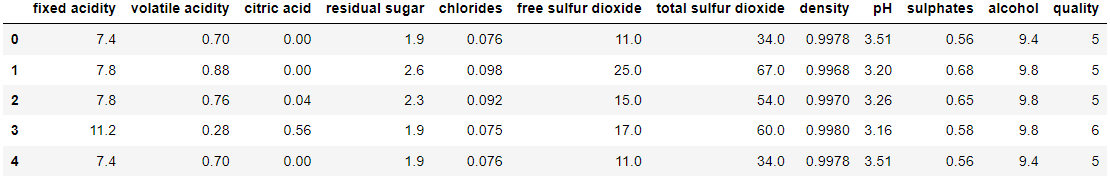As we can see, almost all the parameters have float data type except for quality, which is also our target variable. Since all the independent features are continuous, we might as well learn something from the distributions. A distribution plot depicts the variation in the data distribution. Let’s plot the distribution for each variable using the Seaborn library.

``````fig = plt.figure(figsize = [20,10])
cols = wine_quality.columns
cnt = 1
for col in cols :
plt.subplot(4,3,cnt)
sns.distplot(wine_quality[col],hist_kws=dict(edgecolor="k", linewidth=1,color='blue'),color='red')
cnt+=1
plt.tight_layout()
plt.show()
``````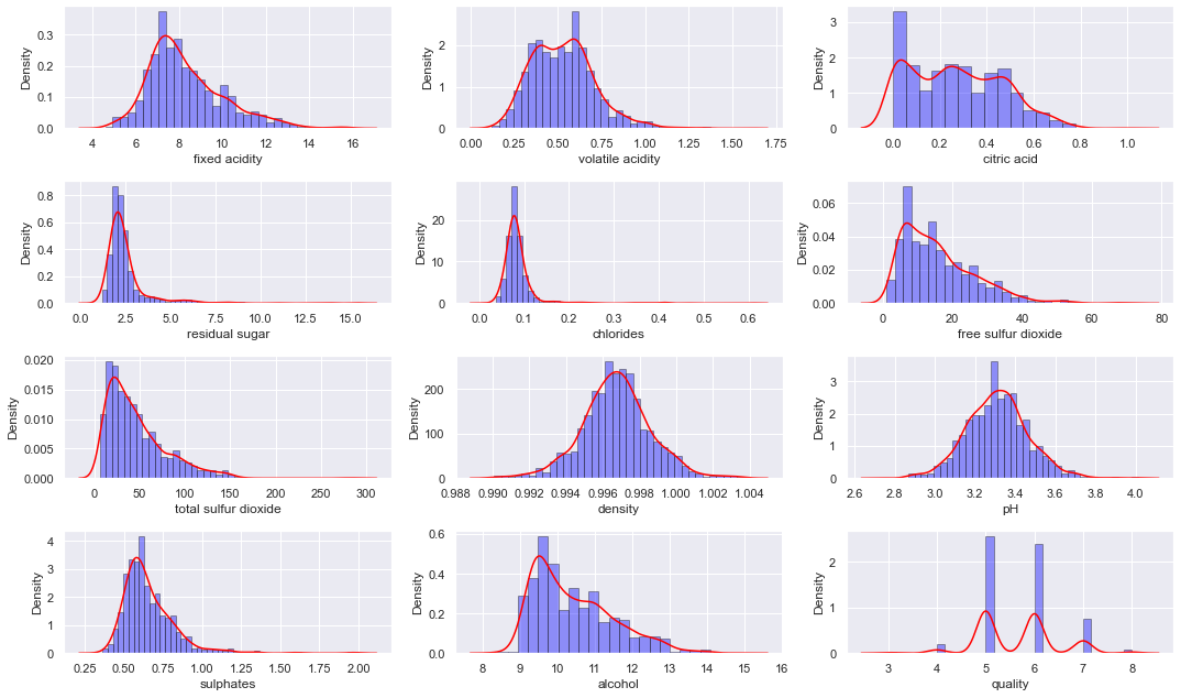The majority of the distributions are approximately normal, while others have some skewness. The wine quality scores 5 and 6 are frequent than others.

#### Interdependency of parameters

We also need to understand the inter-dependency of parameters over each other. A correlation plot would be helpful in the visualization of such dependencies. Let’s plot the correlation heat map for understanding the dependencies!

``````cmap = sns.diverging_palette(500, 10, as_cmap=True)
sns.heatmap(wine_quality.corr(), cmap=cmap, center=0, square=True)
``````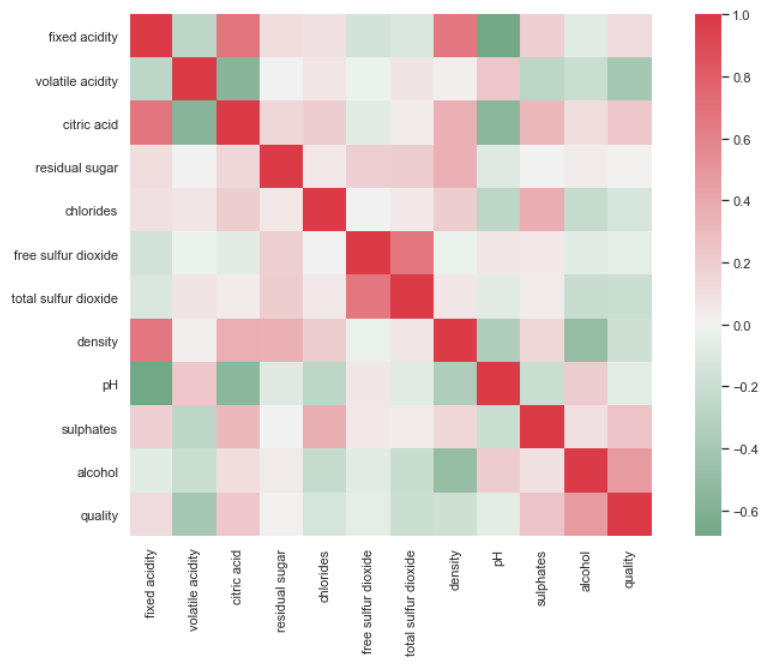From the above correlation heat-map, we can infer that the wine quality is positively correlated with the alcohol content and sulfates. On the contrary, Volatile acidity has a considerable negative correlation with Volatile acidity. It is reasonable that a lower level of acidity is favored in quality testings.

Let’s confirm the above-mentioned relationship using the reg-plots.

``````fig, axs = plt.subplots(nrows=1, ncols=3, figsize=(20,5))
cols = ['volatile acidity', 'alcohol', 'sulphates']
for col, ax in zip(cols, axs.flat):
sns.regplot(x = wine_quality[col],y = wine_quality["quality"], color = 'purple', ax=ax)
``````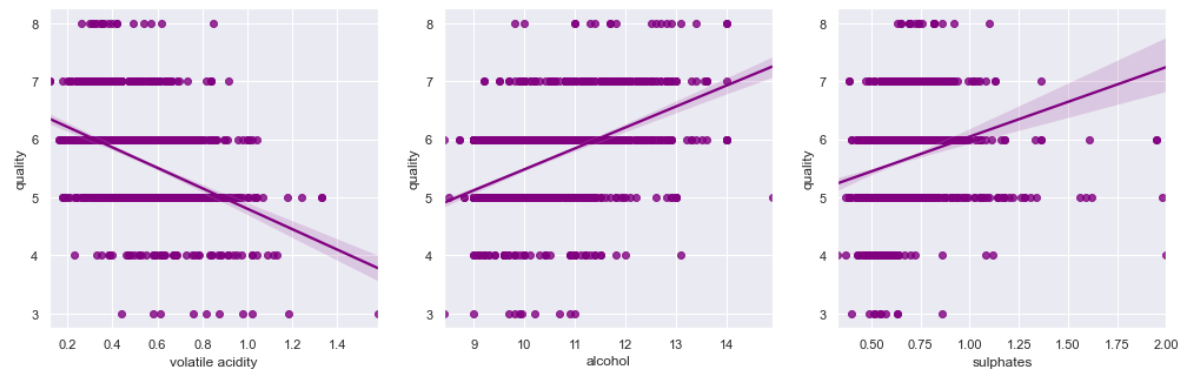Wine Quality has a confirmed negative relationship with Volatile acidity and a positive relationship with alcohol and sulfates.

Let’s dive deeper into the details by visualizing the dependency between Wine Quality and our numeric variables of interest (Independent Feature).

``````cols = wine_quality.columns
cnt = 1
for col in cols :
plt.subplot(4,3,cnt)
sns.boxplot(x="quality",y=col,data=wine_quality,palette="coolwarm")
cnt = cnt + 1
plt.show()
``````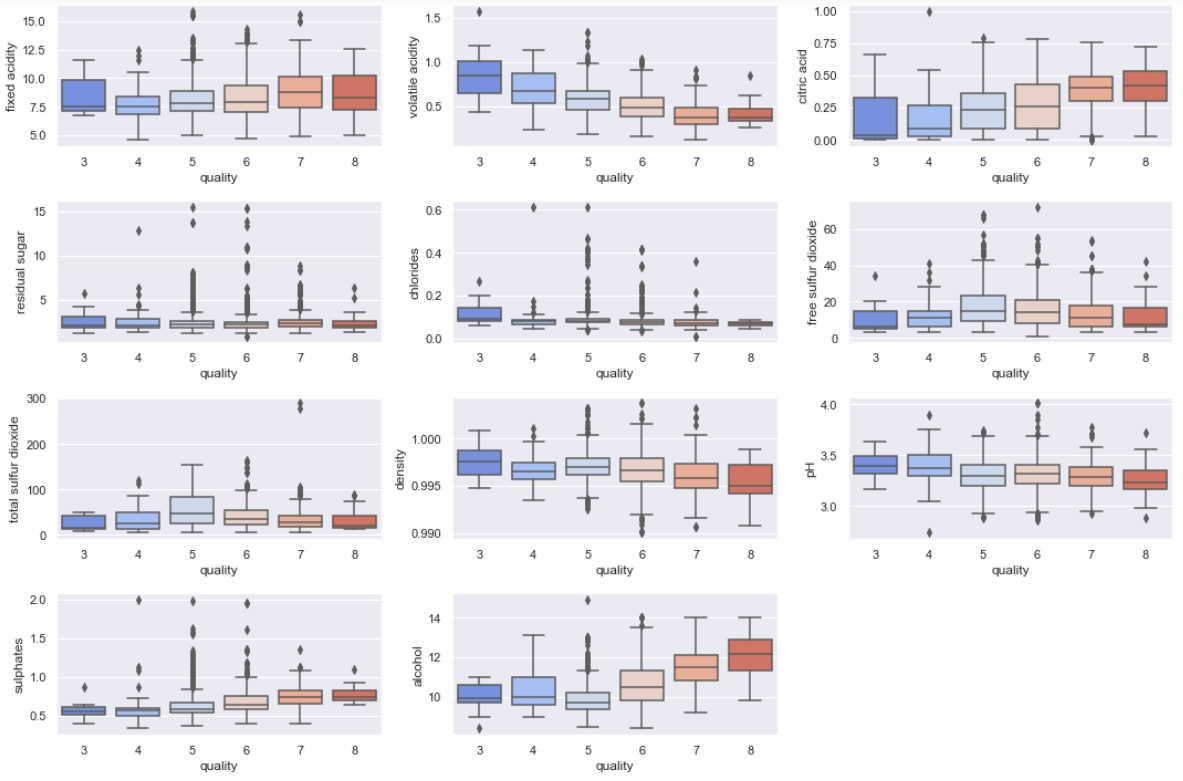Let’s summarise our findings from the above boxplots:

• Highly Rated Wines has comparatively higher Alcohol, Citric Acid, and Sulphates.
• On the contrary, Wines with high volatile acidity, density, and pH are low in quality.
• Wines Quality has no significant relationship with total sulfur dioxide, free sulfur dioxide, chlorides, residual sugar, and fixed acidity.

#### Model Building

Assessing a wine manually is a tedious task and requires an experienced practitioner to evaluate the quality. We will address this problem by building a regression model that will take wine parameters' input and return a predicted quality score. Through this approach, we aim to eliminate the manual tasting and scoring process. To accomplish this task, we need to select a regression algorithm that suffices our requirements.

Following are some regression algorithms that can be used for predicting The Red Wine Quality.

• Linear Regression
• Decision Tree Regressor
• Support Vector Regressor
• k-NN Regressor
• Random Forest Regressor

Linear Models are relatively less complex and explainable, but linear models perform poorly on data containing the outliers. Also, linear models fail to perform well on non-linear datasets. In such cases, non-linear regression algorithms Random Forest Regressor and XGBoost Regressor perform better in fitting the nonlinear data.

Which algorithm is best suited for our use case?

We don’t have significant outliers in this data, indicating that we can use linear as well as complex models. However, the model should have the following qualities:

• Simple and explainable
• Provides accurate predictions
• Robust to concept drift and outliers

Keeping all the above-mentioned points in mind, we need to select a regression model. For this tutorial, we will be using the k-NN Regressor.

#### K Nearest NeighborsRegressor

k-NN Algorithm (K Nearest Neighbors) is a supervised machine learning algorithm that can solve classification and regression tasks. It is extensively used in statistical estimations and even in pattern recognition during the early 1970s. The k-NN algorithm uses feature similarity to predict the values of any new data points. It estimates the value of a data point by taking out the average of ‘K’ closest values in the euclidean space. The most commonly used method for calculating the distance between two data points is known as Euclidean Distance.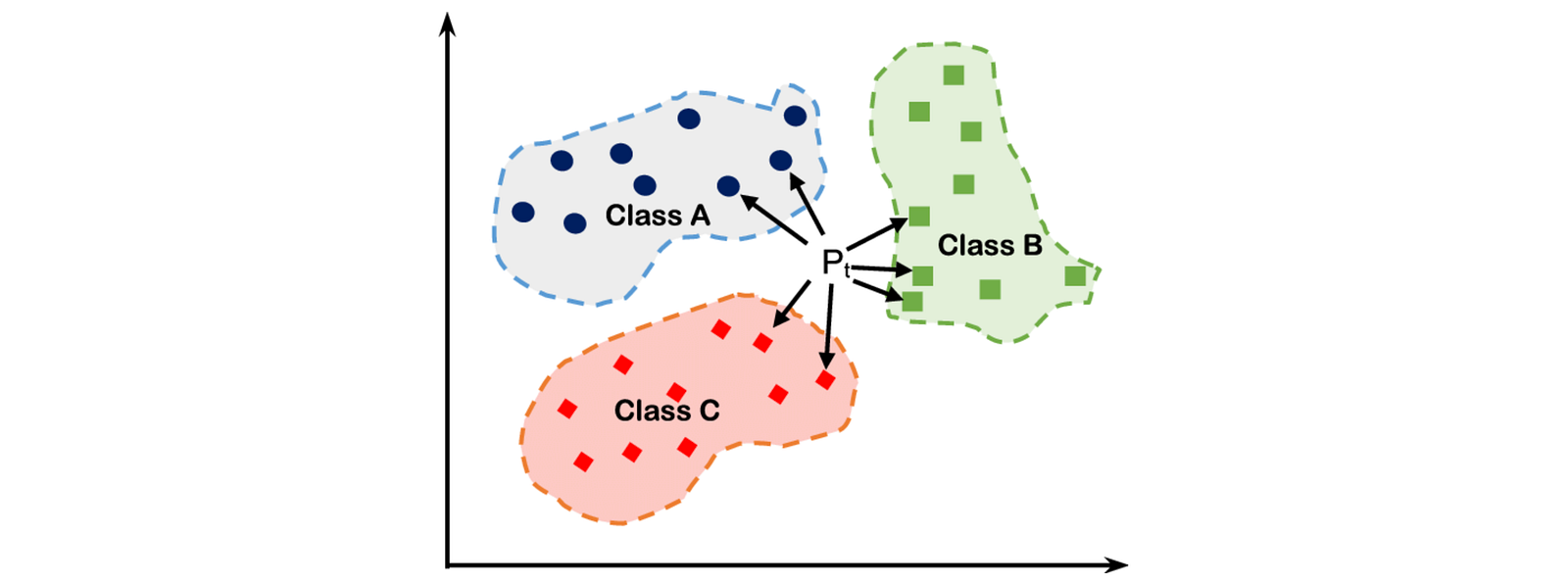Source: Researchgate

Before implementing the k-NN Regressor, we need to scale the features as this algorithm demands homogenous features. We measure the distance between the pair of samples, which are influenced by the measurement units. To avoid this, we should normalize the data before implementing k-NN.

This algorithm has only one hyperparameter, the value of K, which indicates the count of samples that will be treated as the nearest neighbors. One way to find the optimum value of K is to derive a plot between the error obtained on the test set and K denoting values. Finally, choose the K corresponding to the minimum error rate.

Let’s implement the k-NN Regressor:

``````from sklearn import neighbors
from sklearn.preprocessing import MinMaxScaler
from sklearn.model_selection import train_test_split
from sklearn.metrics import mean_squared_error
target = wine_quality['quality']
features = wine_quality.drop('quality', axis = 1)
X_train, X_test, Y_train, Y_test = train_test_split(features, target, test_size=0.3)
scaler = MinMaxScaler(feature_range=(0, 1))
X_train = scaler.fit_transform(X_train)
X_train = pd.DataFrame(X_train)
X_test = scaler.fit_transform(X_test)
X_test = pd.DataFrame(X_test)
rms_error = []
for K in range(1,75):
model = neighbors.KNeighborsRegressor(n_neighbors = K)
model.fit(X_train, Y_train)
pred = model.predict(X_test)
error = mean_squared_error(Y_test, pred, squared=False)
rms_error.append(error, K)
x = np.linspace(1,75, num = 75)
y = rms_error
fig, ax = plt.figure(figsize = [8,5])
ax.plot(x,y)
annot_optimum(x,y)
plt.xlabel('K - Values')
plt.ylabel('RMSE Error')
plt.show()
``````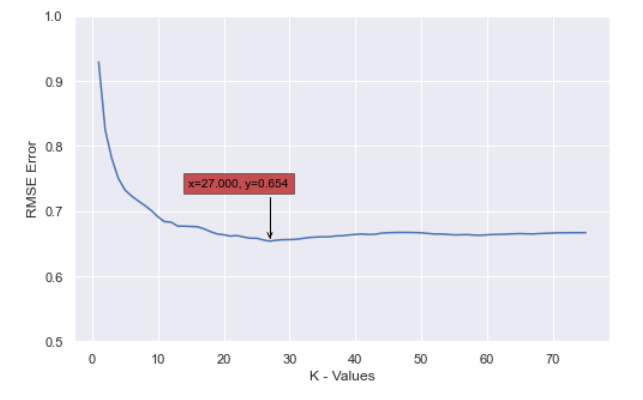We found an optimum Model at K = 27 where the RMS Error is minimum. Our dataset was quite small, which enabled us to find the optimum K; As the dataset grows, the speed of the k-NN algorithm declines very fast, which is a limitation of this algorithm.

#### Pros and Cons of KNN Regressor

Pros:

• Intuitive and Simple
• Only one hyper-parameter to tune
• Efficient method for small datasets.
• Constantly Improves with new training data
• Can be used for both Classification & Regression

Cons:

• Requires feature scaling
• Sensitive to Outliers
• No Capability to handle missing values
• Requires higher space complexity as well as higher time complexity

#### Application of K Nearest Neighbors Algorithm

HEINEKEN N.V

Heineken is second the second largest producer of Beer in the world. However, they also own Zoetermeer Winery for wine production. Heineken relies on Regression analysis to keep track of the quality of the wine. As discussed above, even professional wine tasters are just 71% accurate in determining the quality of Wine.

GRAMMARLY, INC.

Grammarly is a cross-platform cloud-based writing assistant that reviews spelling, grammar, punctuation, clarity, engagement, and delivery mistakes. For categorizing similar sentences and textual documents, Grammarly relies on the KNN classification algorithm.

NETFLIX

Netflix uses the KNN Algorithm to categorize similar content-based shows. Netflix also uses KNN as a Recommendation Engine to recommend similar items; they compare the set of users who like each item — when a similar set of users like two different items, the items themselves are similar!

#### Possible Interview Question

Based on this project, the following questions can be asked in any machine learning interview:

1. Is this problem statement a classification or regression problem?
2. What modifications do we need to do to change regression to classification and vice-versa?
3. Why k-NN? How did you decide the value of k?
4. Why is k-NN very slow? Can we improve the performance of k-NN?
5. What is the evaluation metric for your model? Did you compare your results with existing techniques?

#### Conclusion

We started with a brief introduction to Wine Quality Tasting and problems in the manual Wine tasting approach. Moving on, we discussed the impact of each parameter and started the data analysis. Based on the correlation heat-map, we found out the most significant parameters. We further confirmed their impact on Wine Quality using Boxplots and Regplots. Finally, we built a K-Nearest Neighbors regression model to predict the Quality of Wine and looked at the pros and cons of using the k-NN Regressor model. We could have approached this problem as a Multiclass classification task.

#### Enjoy Learning! Enjoy Algorithms!

Get well-designed application and interview centirc content on ds-algorithms, machine learning, system design and oops. Content will be delivered weekly.